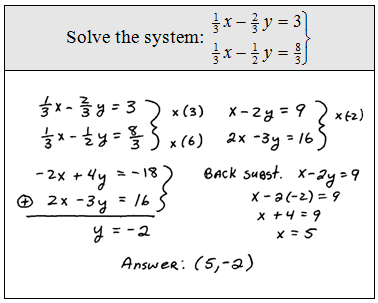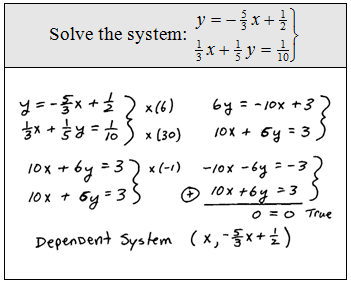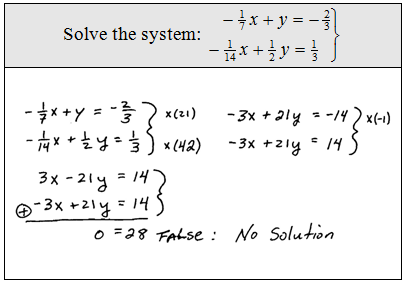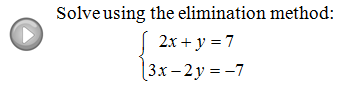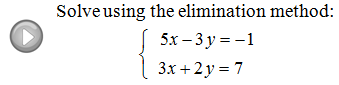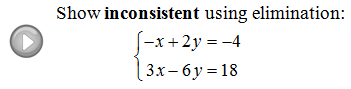## Algebra

Showing posts with label elimination. Show all posts
Showing posts with label elimination. Show all posts

### Solving Linear Systems by Elimination

When solving linear systems, the elimination method - sometimes called the addition method - usually is the method of choice.  This technique is completely algebraic and quick once you get the hang of it.  The idea is to eliminate one variable by adding equivalent equations together.

Solve the system using the elimination method:Step 1: Multiply one or both of the equations by factors that will line up one variable to eliminate.Step 2: Add the equations together.Step 3: Back substitute and present the answer as an ordered pair.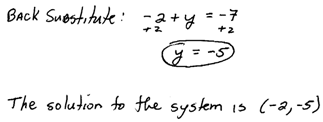Tip: If you multiply an equation by any number - remember to distribute!

Solve the system using the elimination method: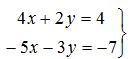To eliminate the variable y, multiply the first equation by 3 and the second equation by 2.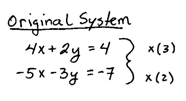You will always have to back substitute to find the value of the other coordinate.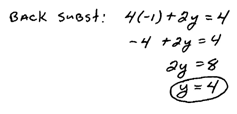Solve the systems using the elimination method: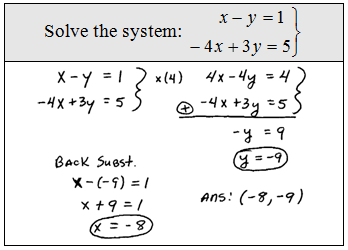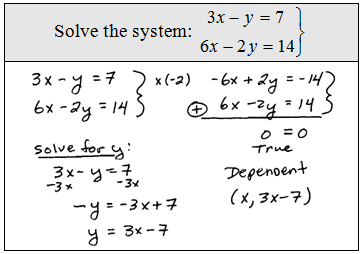You will likely encounter systems that are not lined up in standard form.  In this case, you should first rearrange the equation before using the elimination method.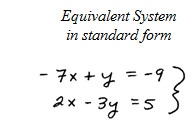Clearing Fractions: If we are given an equation with fractional coefficients, we can clear them out by multiplying both sides by a common multiple of the denominator.  This is a handy technique which we will use often in our study of Algebra.  Do not abuse this, as it only works on equations and not expressions.The LCM of the denominators is 30.Distribute and then simplify.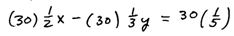No more fractions; now that is nice!This gives equivalent equations.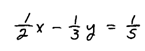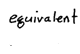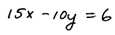Multiply both sides of any equation by the LCD to clear the fractional coefficients.

Solve the systems using the elimination method.